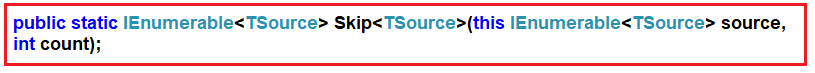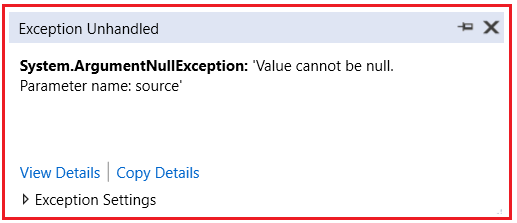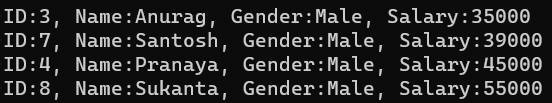# LINQ Skip Method in C#

## LINQ Skip Method in C# with Examples

In this article, I am going to discuss the LINQ Skip Method in C# with Examples. Please read our previous article where we discussed the LINQ TakeWhile Method in C# with Examples. As part of this article, we are going to discuss the following pointers which are related to the LINQ Skip Method.

1. What is LINQ Skip Method?
2. Examples to Understand Skip Method using both Method and Query Syntax.
3. Using the Skip Method with the Where Filtering Method.
4. What happens When we Applied the Filtering Method after the Skip Method in C#?
5. Example when the Data source is null.
##### What is LINQ Skip Method in C#?

The LINQ Skip Method in C# is used to skip or bypass the first n number of elements from a data source or sequence and then returns the remaining elements from the data source as output. Here n is an integer value passed to the Skip method as a parameter. There is only one version available for the LINQ Skip method whose signature is shown below.As you can see in the above signature, the LINQ Skip method takes one integer count parameter and this method going to skip that number of elements from the beginning of the data source. If the data source is null then it is going to throw an exception. We can use the LINQ Skip method with both Method and Query Syntax. The most important point that you need to remember is that it will not make any changes to the positions of the elements in the original data source.

##### Examples to Understand LINQ Skip Method in C#:

Let us see an example to understand the LINQ Skip Method in C# using both Method and Query Syntax. Please have a look at the following example. In the following example, we created one integer collection which contains 10 elements. Here, we need to skip the first four elements and then we need to retrieve the remaining elements using the LINQ Skip method. There is no such operator called skip available in LINQ to write the query syntax, So, here we need to use mixed syntax. The following example code is self-explained, so please go through the comment lines for a better understanding.

```using System;
using System.Collections.Generic;
using System.Linq;
namespace LINQSkipMethodDemo
{
class Program
{
static void Main(string[] args)
{
//Data Source
List<int> numbers = new List<int>() { 1, 2, 3, 4, 5, 6, 7, 8, 9, 10 };

//Skip the First Four Elements and Return Remaining Elements from the Data Source

//Using Method Syntax
List<int> ResultMS = numbers.Skip(4).ToList();

//Using Mixed Syntax
List<int> ResultQS = (from num in numbers
select num).Skip(4).ToList();

//Accessing the Elements using a Foreach Loop
foreach (var num in ResultMS)
{
Console.Write(\$"{num} ");
}

}
}
}
```

Output: 5 6 7 8 9 10

##### LINQ Skip Method with Filtering Method in C#:

Now, let us understand how we can use the LINQ Skip method along with LINQ Where Extension Method and what is the better approach to use these two methods. Our requirement is to skip the first four elements and returns the remaining elements from the collection but the condition is that the elements should be greater than 3. In such cases, first, we need to filter the data using the LINQ Where Extension Method and then we need to skip the first four elements using the LINQ Skip Method. For a better understanding, please have a look at the following example.

```using System;
using System.Collections.Generic;
using System.Linq;
namespace LINQSkipMethodDemo
{
class Program
{
static void Main(string[] args)
{
//Sequence Contains 10 Elements
List<int> numbers = new List<int>() { 1, 2, 3, 4, 5, 6, 7, 8, 9, 10 };

//Skipping the First four elements and Return Remaining Elements from the Sequence
//where Number > 3
//Using Method Syntax
List<int> ResultMS = numbers.Where(num => num > 3).Skip(4).ToList();

//Using Query Syntax
List<int> ResultQS = (from num in numbers
where num > 3
select num).Skip(4).ToList();

//Accessing the Results using Foreach Loop
foreach (var num in ResultMS)
{
Console.Write(\$"{num} ");
}

}
}
}```

Output: 8 9 10

##### Applying Filtering After the Take Method in C#:

If you apply the Filtering Method i.e. the Where Method after the Skip method then you will not get the result as expected. For a better understanding, please have a look at the following example and see the output. Our requirement is to skip the first four elements and return the remaining elements with the condition that the number should be greater than 4. So, our expected output will be 8, 9, and 10.

```using System;
using System.Collections.Generic;
using System.Linq;
namespace LINQTakeMethodDemo
{
class Program
{
static void Main(string[] args)
{
//Sequence Contains 10 Elements
List<int> numbers = new List<int>() { 1, 2, 3, 4, 5, 6, 7, 8, 9, 10 };

//Skipping the First three elements and Returns the Remaining Elements
//from the Sequence where Number > 4

//Using Method Syntax
List<int> ResultMS = numbers.Skip(3).Where(num => num > 4).ToList();

//Using Query Syntax
List<int> ResultQS = (from num in numbers
select num)
.Skip(3)
.Where(num => num > 4)
.ToList();

//Accessing the Results using Foreach Loop
foreach (var num in ResultMS)
{
Console.Write(\$"{num} ");
}

}
}
}
```

Output: 5 6 7 8 9 10

As you can see in the above output, we are getting 5, 6, 7, 8, 9, and 10 instead of 8, 9, and 10. This is because of the wrong use of the Where Extension Method. In this case, first, the Skip method is executed which will skip the first three elements and return the remaining seven elements, and then on this result set the Where Extension method is executed which will further filter the data based on the specified condition. So, it is always a good programming practice to use the Where Method first and then the Skip Method.

##### What happens when the Data Source is null?

When we are applying the Skip Method on a data source that is null, then we will get an exception i.e. ArgumentNullException as shown in the below example.

```using System;
using System.Collections.Generic;
using System.Linq;
namespace LINQTakeMethodDemo
{
class Program
{
static void Main(string[] args)
{
//Sequence is Null
List<int> numbers = null;

//Skip the First three elements and Returns the Remaining Elements

//Using Method Syntax
List<int> ResultMS = numbers.Skip(3).ToList();

//Using Query Syntax
List<int> ResultQS = (from num in numbers
select num)
.Skip(3)
.ToList();

//Accessing the Results using Foreach Loop
foreach (var num in ResultMS)
{
Console.Write(\$"{num} ");
}

}
}
}
```

When you run the application, you will get the following exception.##### Example to Understand LINQ Skip Extension Method with Complex Types in C#:

Let us see how to use the LINQ Skip Extension Method with Complex Data Types using C#. We are going to use the following Employee class. So, create a class file with the name Employee.cs and then copy and paste the following code into it. As you can see, here we created the Employee class with four properties i.e. ID, Name, Gender, and Salary. Here, we have also created one method which will return the list of all employees which will be going to our data source.

```using System.Collections.Generic;
namespace LINQSkipMethodDemo
{
public class Employee
{
public int ID { get; set; }
public string Name { get; set; }
public string Gender { get; set; }
public int Salary { get; set; }

public static List<Employee> GetAllEmployees()
{
return new List<Employee>()
{
new Employee { ID = 1, Name = "Preety", Salary = 10000, Gender = "Female"},
new Employee { ID = 2, Name = "Priyanka", Salary =20000, Gender = "Female"},
new Employee { ID = 3, Name = "Anurag", Salary = 35000, Gender = "Male"},
new Employee { ID = 4, Name = "Pranaya", Salary = 45000, Gender = "Male"},
new Employee { ID = 5, Name = "Sambit", Salary = 15000, Gender = "Male"},
new Employee { ID = 6, Name = "Hina", Salary = 25000, Gender = "Female"},
new Employee { ID = 7, Name = "Santosh", Salary = 39000, Gender = "Male"},
new Employee { ID = 8, Name = "Sukanta", Salary = 55000, Gender = "Male"}
};
}
}
}```
##### Business Requirement: LINQ Skip Method with OrderBy Method

Our Business Requirement is to skip the first four employees who are getting the lowest salary. For this, we need to use the OrderBy method on the data source and we need to sort the employees in ascending order by their salary. Then we need to apply the Skip method and pass the count value as 4 as we need to skip 4 employees. The following example exactly does the same thing.

```using System;
using System.Collections.Generic;
using System.Linq;
namespace LINQSkipMethodDemo
{
class Program
{
static void Main(string[] args)
{
//Data Source
List<Employee> employees = Employee.GetAllEmployees();

//Skip First four Employees who are getting lowest Salary
//Using Method Syntax
List<Employee> ResultMS = employees.OrderBy(emp => emp.Salary).Skip(4).ToList();

//Using Query Syntax
List<Employee> ResultQS = (from emp in employees
orderby emp.Salary ascending
select emp)
.Skip(4)
.ToList();

//Accessing the Results using Foreach Loop
foreach (Employee emp in ResultMS)
{
Console.WriteLine(\$"ID:{emp.ID}, Name:{emp.Name}, Gender:{emp.Gender}, Salary:{emp.Salary}");
}